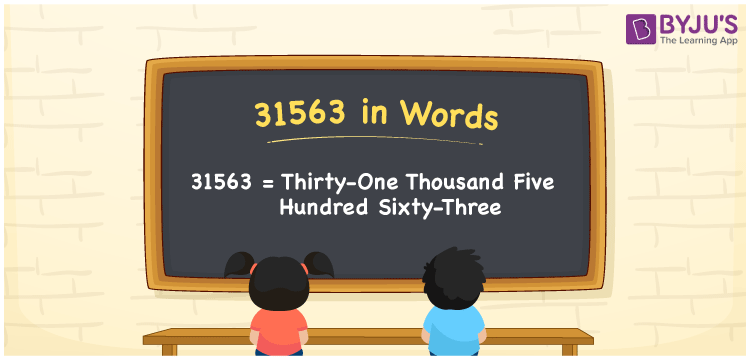# 31563 in Words

31563 in words is written as Thirty-one thousand five hundred sixty-three. In both the International System of Numerals and the Indian System of Numerals, 31563 is written as Thirty-one thousand five hundred sixty-three. The number 31563 is a Cardinal Number as it denotes some quantity. For example, “that motorcycle costs 31563 rupees”.

 31563 in Words Thirty-one thousand five hundred sixty-three Thirty-one thousand five hundred sixty-three in Number 31563

## 31563 in English Words

31563 in English words is read as “Thirty-one thousand five hundred sixty-three”.## How to Write 31563 in Words?

To write 31563 in words, we shall use the place value chart. In the place value chart, put 3 in the ten thousands, 1 in the thousands, 5 in the hundreds, 6 in the tens and 3 in the ones. Let us make a place value chart to write the number 31563 in words.

 Ten Thousands Thousands Hundreds Tens Ones 3 1 5 6 3

Thus, we can write the expanded form as

3 × Ten Thousand + 1 × Thousand + 5 × Hundred + 6 × Ten + 3 × One

= 3 × 10000 + 1 × 1000 + 5 × 100 + 6 × 10 + 3 × 1

= 30000 + 1000 + 500 + 60 + 3

= 31563

= Thirty-one thousand five hundred sixty-three.

31563 is a natural number, the successor of 31562 and the predecessor of 31564.

31563 in words – Thirty-one thousand five hundred sixty-three

• Is 31563 an odd number? – Yes
• Is 31563 an even number? – No
• Is 31563 a perfect square number? – No
• Is 31563 a perfect cube number? – No
• Is 31563 a prime number? – No
• Is 31563 a composite number? – Yes

## Frequently Asked Questions on 31563 in Words

Q1

### How to write 31563 in words?

31563 in words is written as Thirty-one thousand five hundred sixty-three.
Q2

### How to write 31563 in the International and Indian System of Numerals?

In both, the system of numerals, 31563 in words, is written as Thirty-one thousand five hundred sixty-three.
Q3

### How to write 31563 in a place value chart?

In the place value chart, write 3 in the ten thousands, 1 in the thousands, 5 in the hundreds, 6 in the tens and 3 in the ones, respectively.